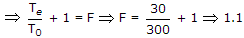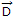# Electronics and Communication Engineering - Electromagnetic Field Theory

46.

Find the noise factor for an antenna at 27°C with equivalent noise temperature 30°K

 A. 20 B. 100 C. 300 D. 1.1

Explanation:

Te = T0(F - 1).

47.

The displacement flux density at a point on the surface of a perfect conductor is= 2(ax - 3 az)c/m2 pointing away from the surface. The charge density at that point c/m2 will be

 A. 2 B. - 2 C. 4 D. - 4

Explanation:

∇ . D = r.

48.

Characteristic impedance of a transmission line is 50 Ω. Input impedance of the open circuited line.
Zoc = 100 + J150 Ω. When transmission line is short circuited, then value of the input impedance will be

 A. 50 Ω B. 100 + J150 Ω C. 7.69 + J11.54Ω D. 7.69 - J11.54 Ω

ZC = ZOC - ZSC50 = (100 + j150)(ZSC) .